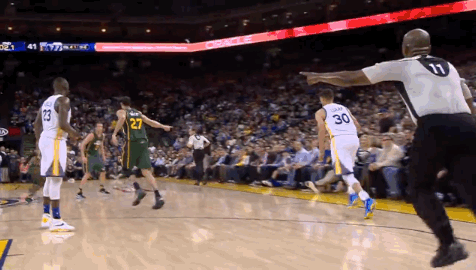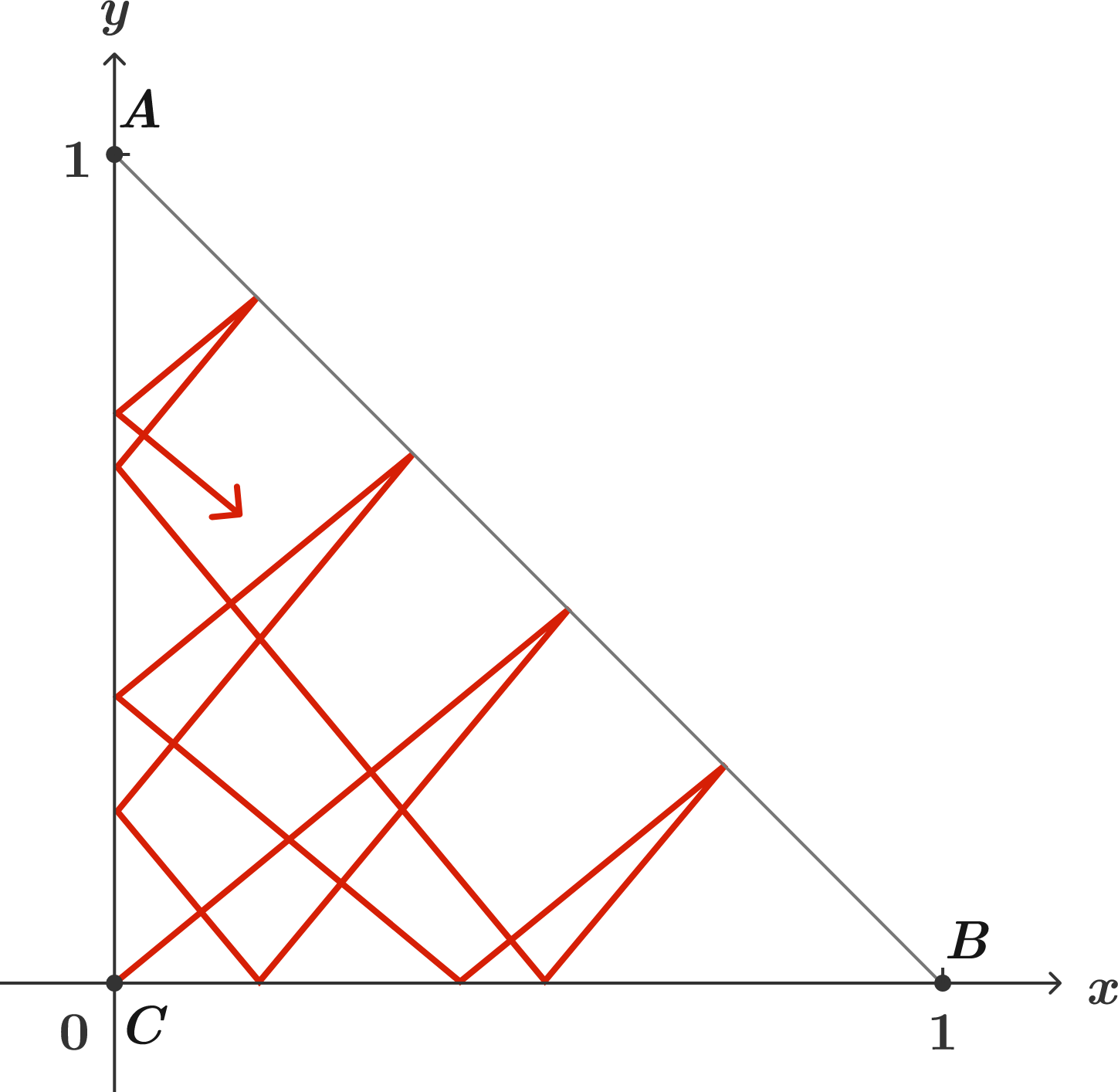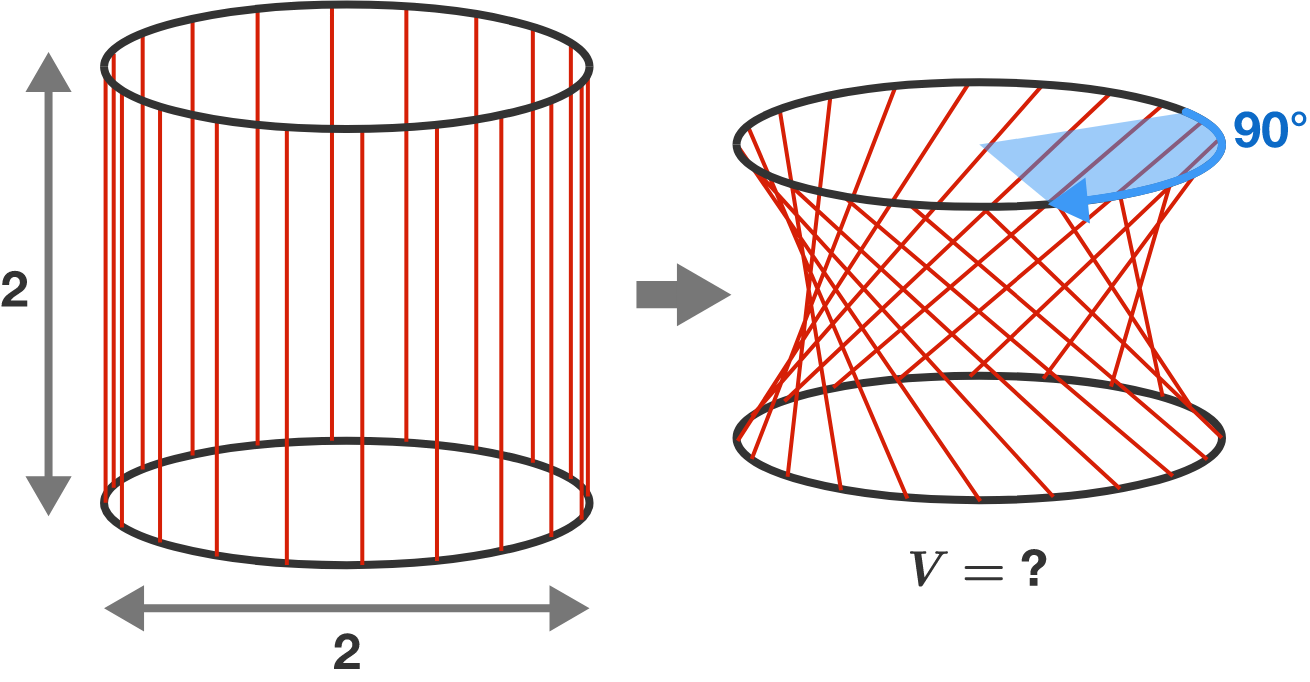Problems of the Week

Contribute a problem

$A$ and $B$ are distinct numbers such that $A^n - B^n$ is an integer for all positive integers $n.$

Do both $A$ and $B$ have to be integers?

Steph Curry regularly wows crowds with his incredible 3-point shooting, as he did in Game 1 of the 2018 NBA championship last Thursday when he made a shot from half-court right at the buzzer.When he shoots, he aims the ball at the center of the rim, horizontal distance $d$ ahead of him and vertical distance $h$ above. There is some freedom in making the shot — there are an infinite number of pairs of shot velocity and shot angle that will result in the ball passing through the target point $(d,h)$. But the key to a consistent shot is minimizing the effect of small errors in execution. In fact, there's a magic angle at which the variation in both $d$ and $h$ with respect to $\theta$ is minimized.

At what angle (in degrees) should he take his shots for the most reproducible three-pointer?

Assumptions and Details:

• $d = \SI{14.5}{\meter}$ and $h = \SI{1.15}{\meter}.$
• Neglect air resistance.
• In general, a player needs to be consistent in their shot angle and shot velocity but, for simplicity, we focus on shot angle and assume that Curry can set his shot velocity with no error.

$f(x) = x+\sin(x).$

What is the area of one of the infinitely many closed regions between the curves $y = f(x)$ and its inverse function $y=f^{-1}(x)?$Triangle $ABC$ has vertices $A = (0,1),$ $B=(1,0),$ and $C=(0,0)$ in the coordinate plane.

The insides of the sides are lined with mirrors, and then a laser beam is fired from the origin with a slope of $\frac{314}{379}.$

Which corner of the triangle will the laser beam hit first?

Two unit circles are joined by many equally spaced strings of length $2$ to form a cylinder.

The upper circle is rotated by $90$ degrees while the lower is fixed. If there were infinitely many strings, they would serve as the surface of a 3D figure, together with the two circles.

What is the volume inside this 3D figure?×